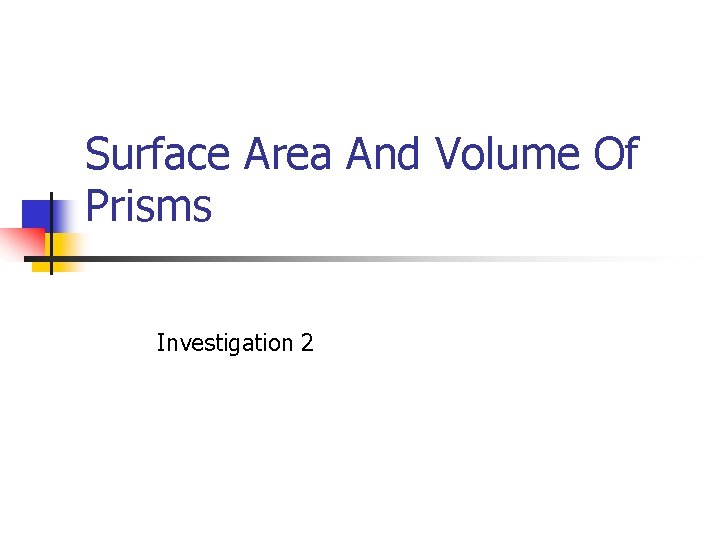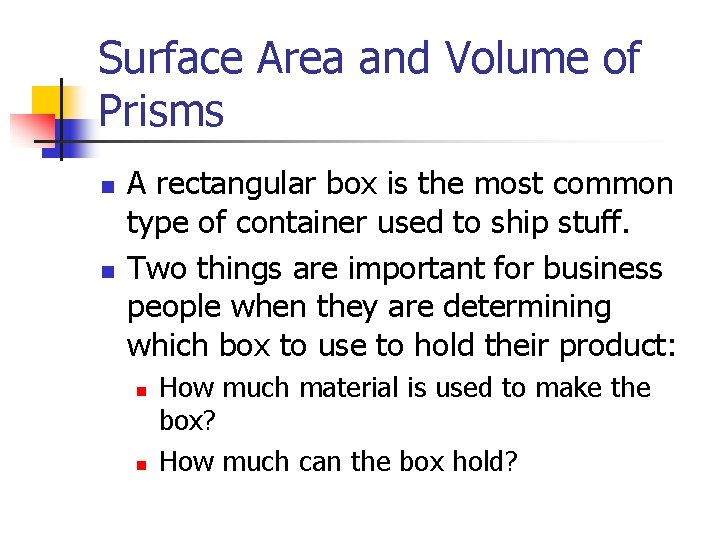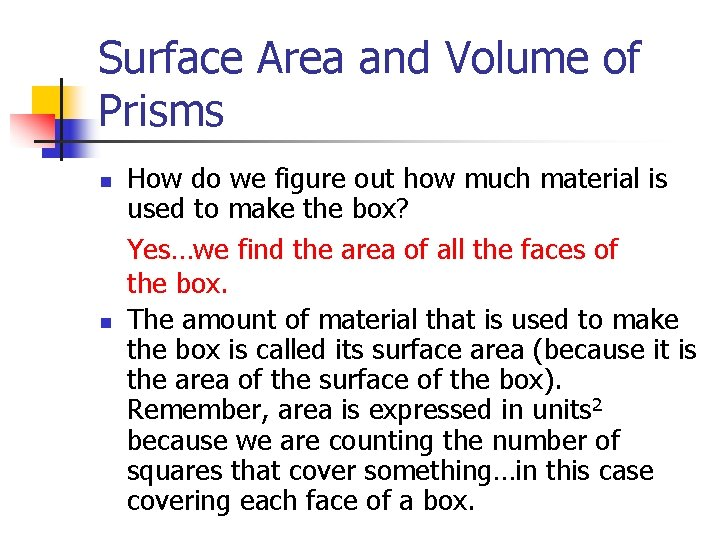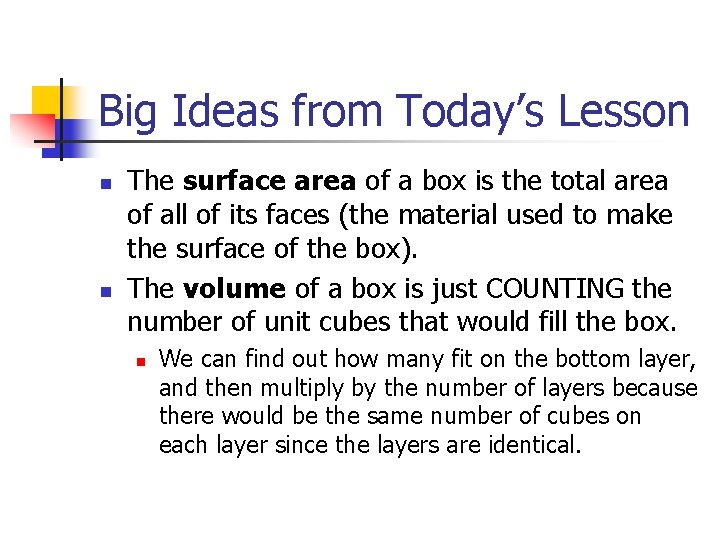# Surface Area And Volume Of Prisms Investigation 2

• Slides: 9Surface Area And Volume Of Prisms Investigation 2Today’s Learning Goal n n We will continue to work with flat patterns of prisms to help us see the total area of the 3 -D shape. We will continue to think about how to find the volume of prisms.Surface Area and Volume of Prisms n n A rectangular box is the most common type of container used to ship stuff. Two things are important for business people when they are determining which box to use to hold their product: n n How much material is used to make the box? How much can the box hold?Surface Area and Volume of Prisms n n How do we figure out how much material is used to make the box? Yes…we find the area of all the faces of the box. The amount of material that is used to make the box is called its surface area (because it is the area of the surface of the box). Remember, area is expressed in units 2 because we are counting the number of squares that cover something…in this case covering each face of a box.Surface Area and Volume of Prisms n n How do we figure out how much a box can hold? Yes…we find out how many unit cubes can fit inside the box. The number of unit cubes that can fit inside a box is called its volume. Volume is expressed in cubic units, such as cubic inches or cubic centimeters, because we are counting the number of cubes that fit inside a box. We can abbreviate cubic units by writing units 3. For example, 54 in 3 means 54 cubic inches.Partner Work n You have 20 minutes to work on the following questions with your partner.For those that finish early A number cube is designed so that numbers on opposite sides add to 7. Copy the flat pattern below and write the integers from 1 to 6 on the flat pattern so that it can be folded to form a number cube.Big Ideas from Today’s Lesson n n The surface area of a box is the total area of all of its faces (the material used to make the surface of the box). The volume of a box is just COUNTING the number of unit cubes that would fill the box. n We can find out how many fit on the bottom layer, and then multiply by the number of layers because there would be the same number of cubes on each layer since the layers are identical.Homework n Complete Homework Worksheet